# Math

These helper functions are all based around manipulating data related to numbers.

## Abs

The abs function takes a number as input and gives you the magnitude of that number. Magnitude refers to the distance of a number from zero on a number line, regardless of its positive or negative sign.

For example, if you use the abs function with the number 12012.1000, it will return 12012.1. This means that the magnitude of 12012.1000 is 12012.1, regardless of whether it is positive or negative.The add function takes two numbers, "a" and "b", as input and returns the sum of those two numbers. It simply adds the values of "a" and "b" together.

For example, if you use the add function with the numbers 1 and 2, it will return 3. This means that the sum of 1 and 2 is 3.## Avg

The avg function calculates the average of a given array of numbers. It takes an array as input and returns the average value, which is the sum of all the numbers divided by the total count of numbers in the array.

For example, if you use the avg function with the array `[1, 2, 3, 4, 5]`, it will return `3`. This means that the average of the numbers in the array is `3`. It calculates the sum of all the numbers `(1 + 2 + 3 + 4 + 5 = 15)` and divides it by the total count of numbers `(5)`, resulting in an average of `3`.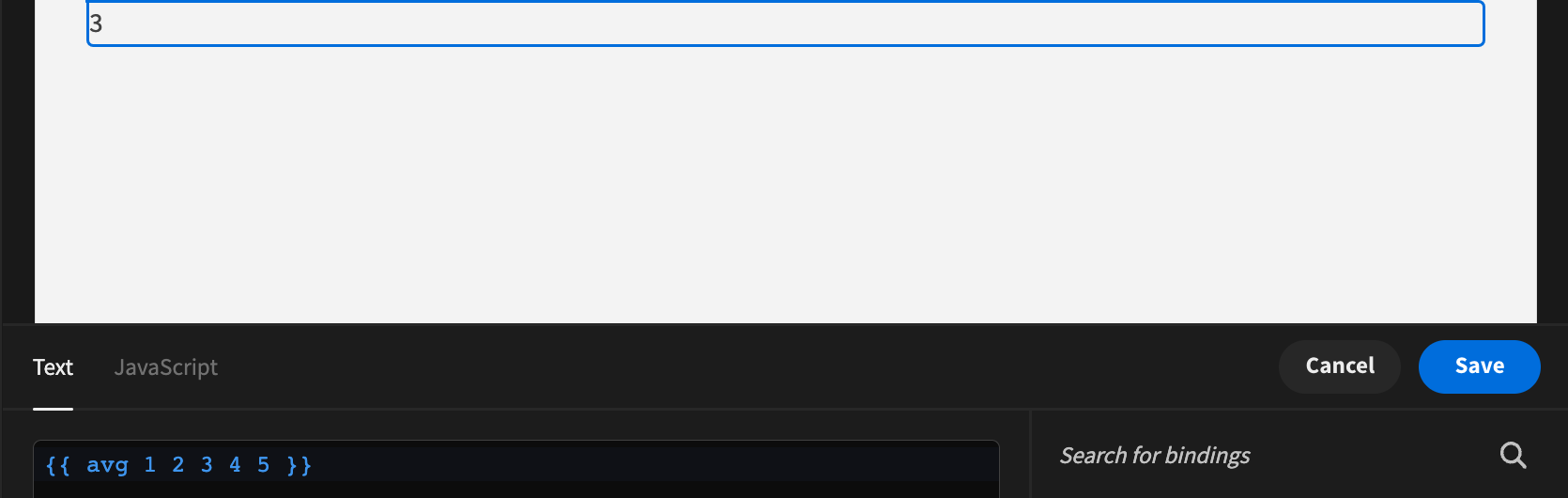## Ceil

The ceil function takes a value as input and returns the smallest integer that is greater than or equal to that value. It uses the Math.ceil() function internally.

For example, if you use the ceil function with the value 1.2, it will return 2. This means that the smallest integer greater than or equal to 1.2 is 2. The ceil function essentially rounds up the value to the nearest whole number.## Divide

The divide function takes two numbers, "a" and "b", as input and returns the result of dividing "a" by "b". It performs the division operation.

For example, if you use the divide function with the numbers 10 and 5, it will return 2. This means that 10 divided by 5 is equal to 2. The divide function essentially calculates the quotient when "a" is divided by "b".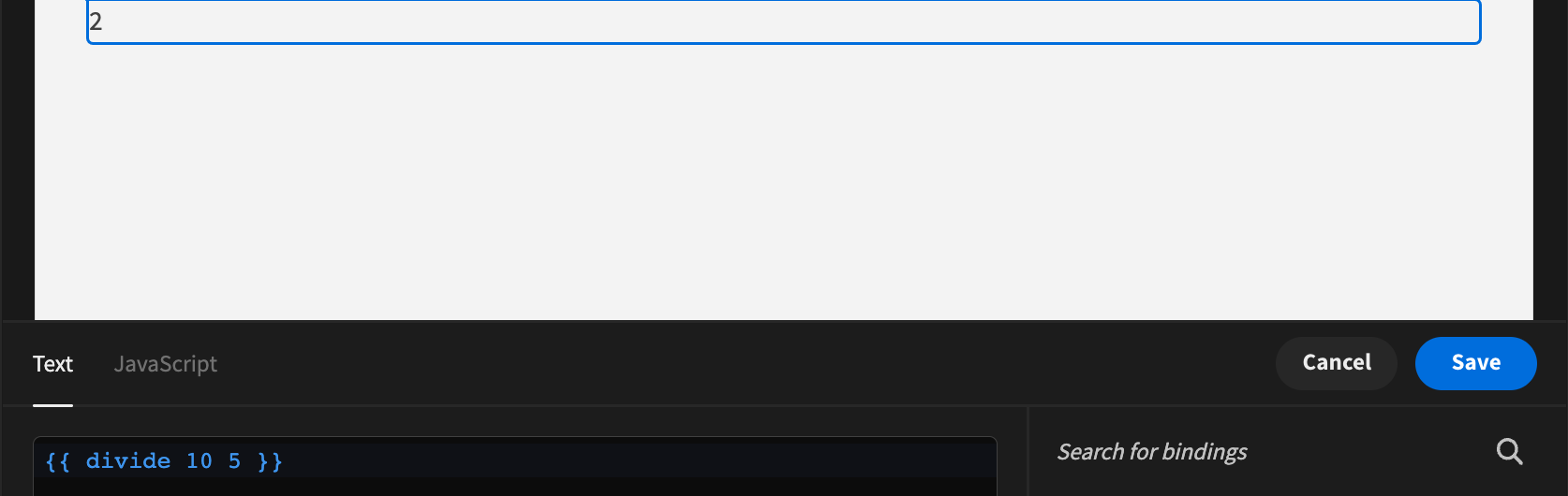## Floor

The floor function takes a value as input and returns the largest integer that is less than or equal to that value. It uses the Math.floor() function internally.

For example, if you use the floor function with the value 1.2, it will return 1. This means that the largest integer less than or equal to 1.2 is 1. The floor function essentially rounds down the value to the nearest whole number.## Minus

The minus function takes two numbers, "a" and "b", as input and returns the result of subtracting "b" from "a". It performs the subtraction operation.

For example, if you use the minus function with the numbers 10 and 5, it will return 5. This means that subtracting 5 from 10 gives you a result of 5. The minus function calculates the difference between "a" and "b".## Modulo

The modulo function takes two numbers, "a" and "b", as input and returns the remainder obtained when "a" is divided by "b". It calculates the remainder of a division operation.

For example, if you use the modulo function with the numbers 10 and 5, it will return 0. This means that when you divide 10 by 5, the remainder is 0. The modulo function essentially gives you the leftover value after performing the division operation.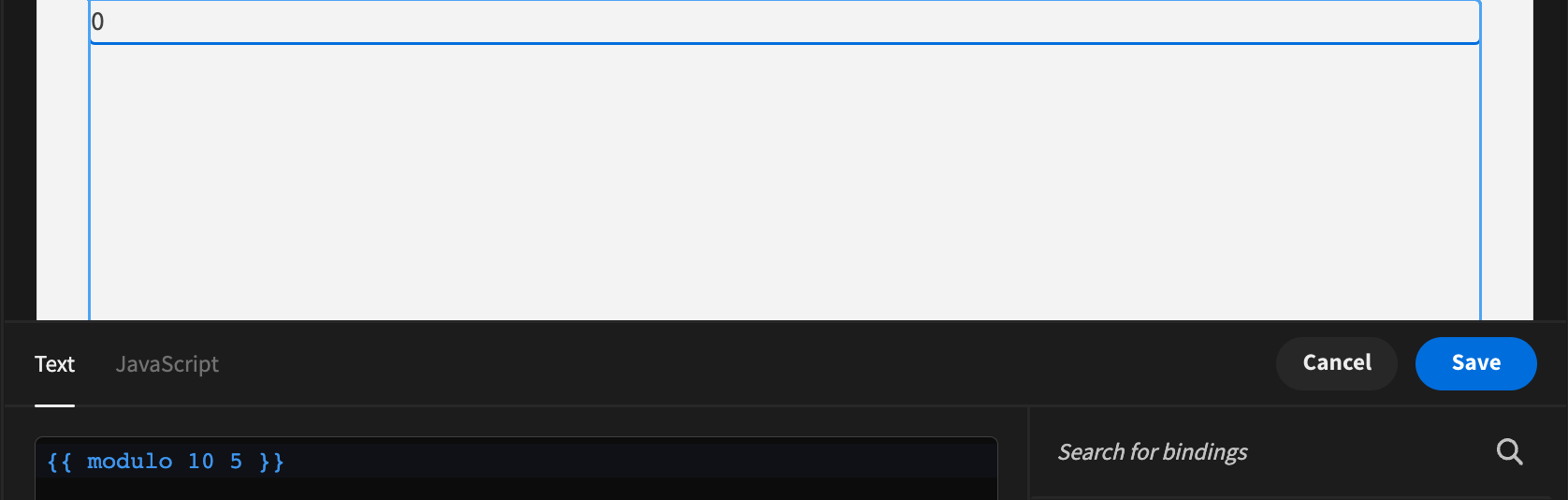## Multiply

The multiply function takes two numbers, "a" and "b", as input and returns the product of the two numbers. It performs the multiplication operation.

For example, if you use the multiply function with the numbers 10 and 5, it will return 50. This means that multiplying 10 by 5 gives you a product of 50. The multiply function calculates the result of multiplying "a" and "b" together.## Plus

The plus function takes two numbers, "a" and "b", as input and returns the sum of the two numbers. It performs the addition operation.

For example, if you use the plus function with the numbers 10 and 5, it will return 15. This means that adding 10 and 5 gives you a sum of 15. The plus function calculates the result of adding "a" and "b" together.## Random

The random function generates a random number between two given values. It takes two arguments, "min" and "max", representing the minimum and maximum values of the desired range.

For example, if you use the random function with the values 0 and 20, it may return 10. This means that a random number between 0 and 20 was generated, and in this particular case, it happened to be 10. The random function provides a way to obtain a random number within a specified range. Please note that each time you call the random function, it may produce a different random number within the given range.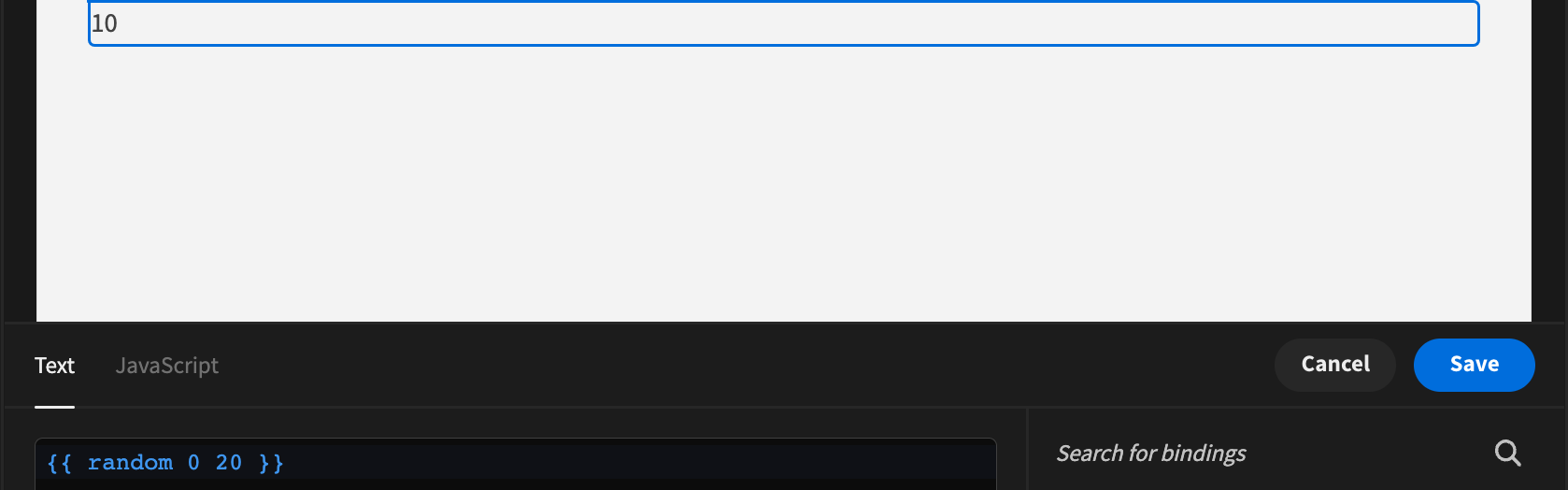## Remainder

The remainder function takes two numbers, "a" and "b", as input and returns the remainder when "a" is divided by "b". It calculates the remainder of the division operation.

For example, if you use the remainder function with the numbers 10 and 6, it will return 4. This means that when you divide 10 by 6, the remainder is 4. The remainder function gives you the leftover value after performing the division operation between "a" and "b".## Round

The round function takes a number as input and returns the rounded value of that number. It performs rounding based on the standard rounding rules.

For example, if you use the round function with the number 10.3, it will return 10. This means that the given number 10.3 was rounded to the nearest whole number, which is 10. The round function adjusts the value to the closest integer based on the decimal portion of the number.## Subtract

The subtract function takes two numbers, "a" and "b", as input and returns the result of subtracting "b" from "a". It performs the subtraction operation.

For example, if you use the subtract function with the numbers 10 and 5, it will return 5. This means that subtracting 5 from 10 gives you a result of 5. The subtract function calculates the difference between "a" and "b".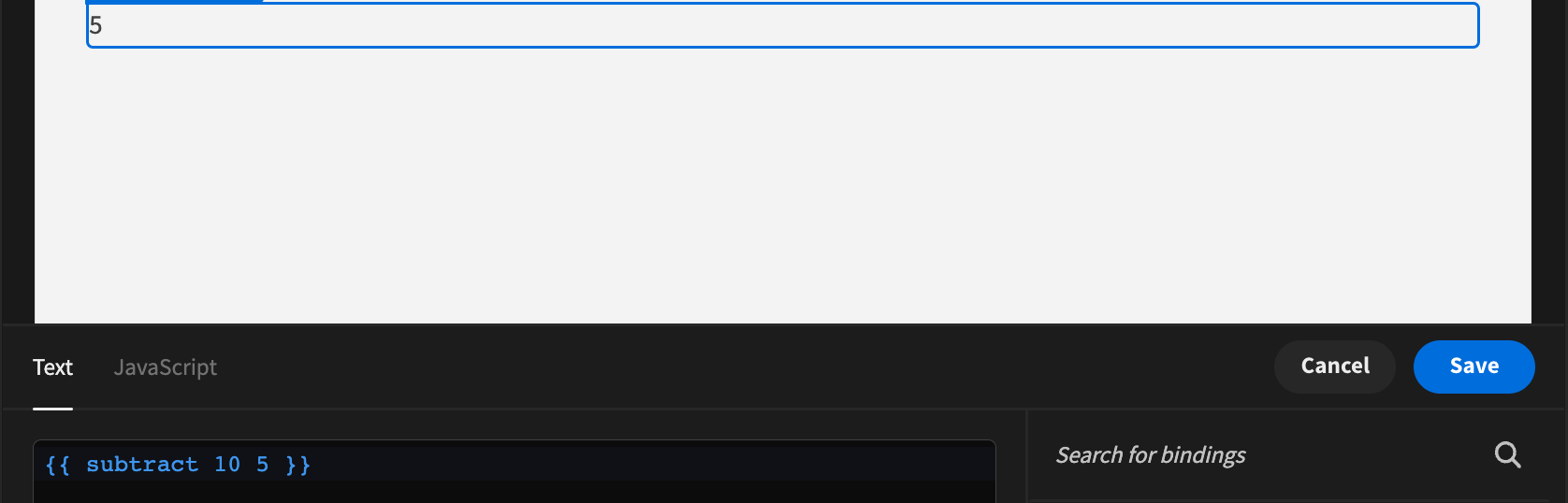## Sum

The sum function takes an array of numbers as input and returns the sum of all the numbers in the array. It calculates the total sum of the elements in the array.

For example, if you use the sum function with the array `[1, 2, 3]`, it will return `6`. This means that the sum of the numbers `1, 2,` and `3 is 6`. The sum function adds up all the numbers in the array to give you the total sum.

What’s Next